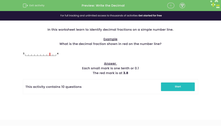# Write the Decimal

In this worksheet, students identify the decimal fractions on a simple number line.Key stage:  KS 2

Curriculum topic:   Number: Number and Place Value

Curriculum subtopic:   Use Different Representations of Numbers

Difficulty level:#### Worksheet Overview

In this worksheet learn to identify decimal fractions on a simple number line.

Example

What is the decimal fraction shown in red on the number line?4